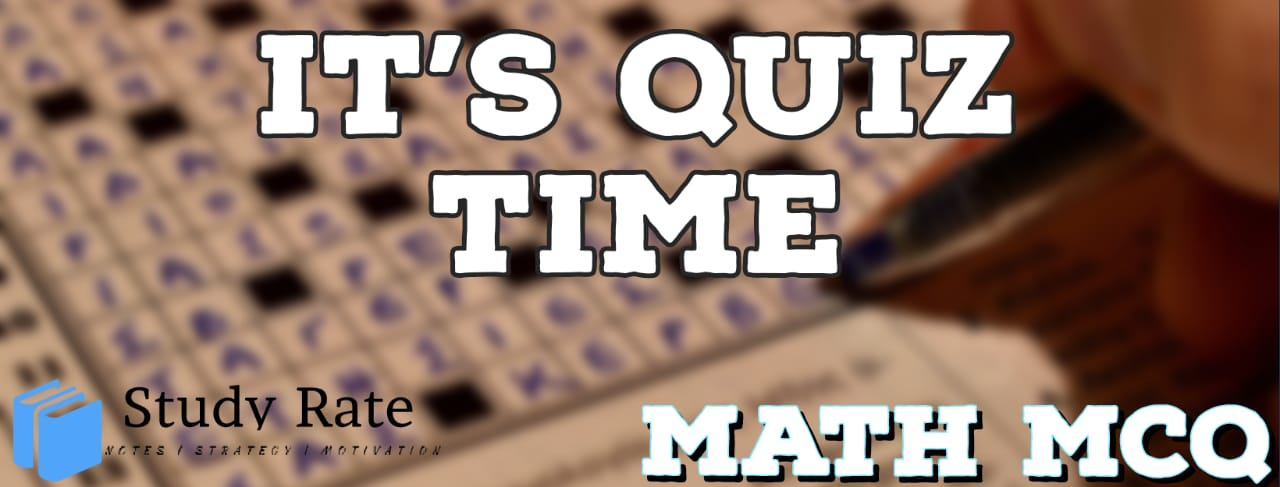## CBSE Class 10 Maths Chapter 2 – Polynomials MCQ Quiz

Free PDF Download of CBSE Class 10 Maths Chapter 2 Polynomials Multiple Choice Questions with Answers. MCQ Questions for Class 10 Maths with Answers was Prepared Based on Latest Exam Pattern. Students can solve Class 10 Maths Polynomials MCQs with Answers to know their preparation level

Polynomials Chapter 2 of Class 10 Maths.

1. The polynomial p(x)=x−323 is a___

2.3. Three curves i.eare depicted in the graph shown below. Which of the polynomials does graph 3 represent?4. According to the graph below, the product of the zeroes of the polynomial will be5. The zeroes of the quadratic polynomialare

6. Number of polynomials having 3 and 7 as zeroes are?

7. If the zeroes of the quadratic polynomial, c ≠ 0 are equal, then

8. The value of p for which the polynomialis exactly divisible by (x – 2) is:

9.  If α and β are zeroes of x2– 4x + 1, then 1/α + 1/β – αβ is

10. In division algorithm when should one stop the division process?
1. When the remainder is zero.
2. When the degree of the remainder is less than the degree of the divisor.
3. When the degree of the quotient is less than the degree of the divisor.

### This Post Has 2 Comments

1.Ali Asghar

Every useful

2.Ali Asghar

Very use ful site